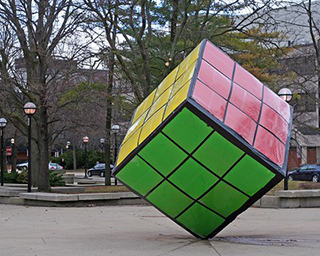18.600 | Fall 2019 | Undergraduate

# Probability and Random Variables

#### Course Description

This course introduces students to probability and random variables. Topics include distribution functions, binomial, geometric, hypergeometric, and Poisson distributions. The other topics covered are uniform, exponential, normal, gamma and beta distributions; conditional probability; Bayes theorem; joint …

## Course Info

##### Learning Resource Types
Problem Sets
Exams with Solutions
Lecture Notes
Online Textbook
Instructor InsightsIn the popular puzzle Rubik’s Cube invented in 1974 by Ernő Rubik, each turn of the puzzle faces creates a permutation of the surface colors. (Image by Kristin on flickr. License CC BY-NC-SA.)